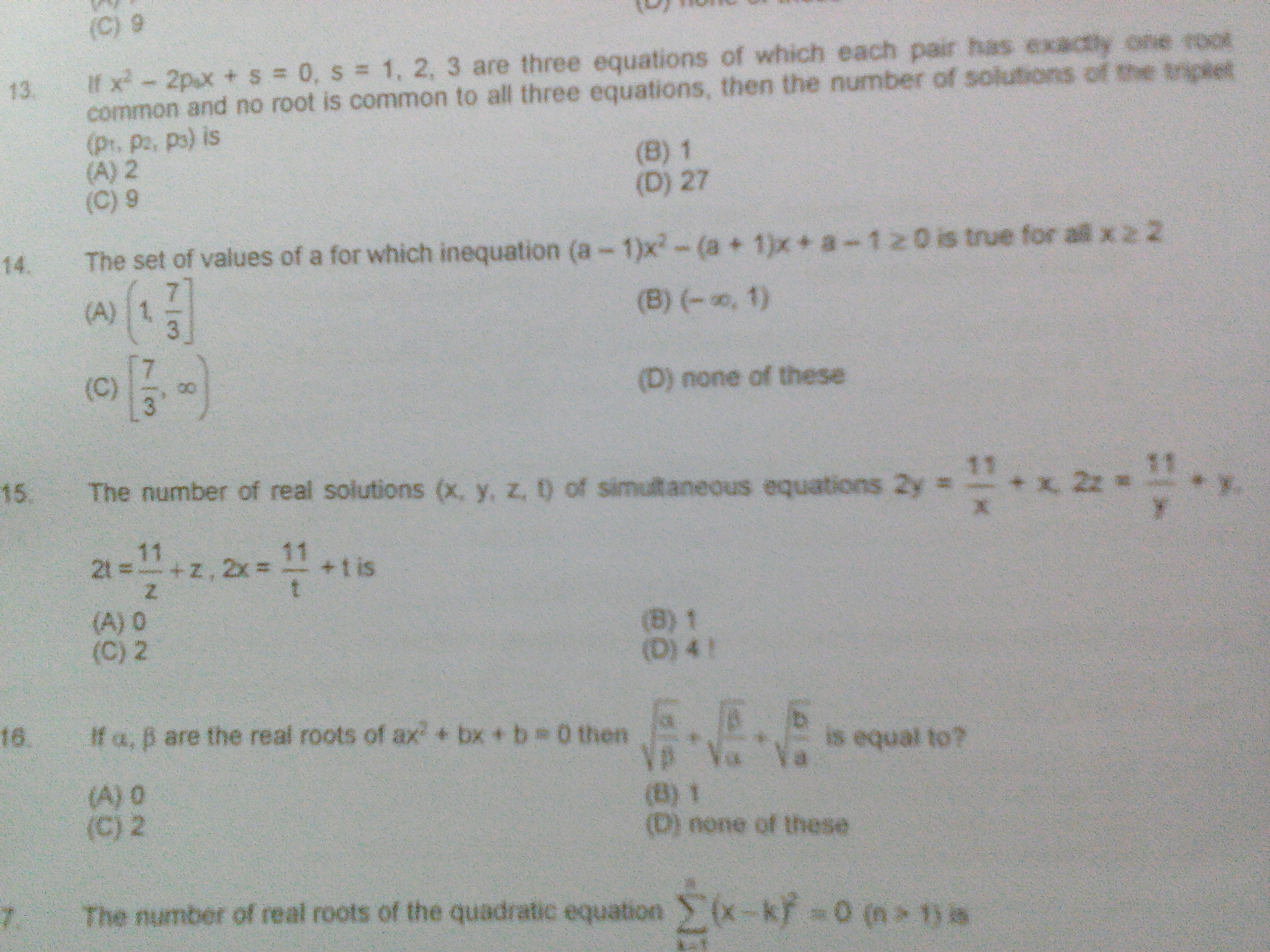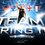# Algebra

Find the number of real solutions $$(x,y,z,w)$$ of the equation below.$x+y+z+w = 11\left(\dfrac{1}{x} + \dfrac{1}{y} +\dfrac{1}{z} +\dfrac{1}{w}\right)$Note by Space Sizzlers
3 years, 7 months ago

This discussion board is a place to discuss our Daily Challenges and the math and science related to those challenges. Explanations are more than just a solution — they should explain the steps and thinking strategies that you used to obtain the solution. Comments should further the discussion of math and science.

When posting on Brilliant:

• Use the emojis to react to an explanation, whether you're congratulating a job well done , or just really confused .
• Ask specific questions about the challenge or the steps in somebody's explanation. Well-posed questions can add a lot to the discussion, but posting "I don't understand!" doesn't help anyone.
• Try to contribute something new to the discussion, whether it is an extension, generalization or other idea related to the challenge.

MarkdownAppears as
*italics* or _italics_ italics
**bold** or __bold__ bold
- bulleted- list
• bulleted
• list
1. numbered2. list
1. numbered
2. list
Note: you must add a full line of space before and after lists for them to show up correctly
paragraph 1paragraph 2

paragraph 1

paragraph 2

[example link](https://brilliant.org)example link
> This is a quote
This is a quote
    # I indented these lines
# 4 spaces, and now they show
# up as a code block.

print "hello world"
# I indented these lines
# 4 spaces, and now they show
# up as a code block.

print "hello world"
MathAppears as
Remember to wrap math in $$ ... $$ or $ ... $ to ensure proper formatting.
2 \times 3 $2 \times 3$
2^{34} $2^{34}$
a_{i-1} $a_{i-1}$
\frac{2}{3} $\frac{2}{3}$
\sqrt{2} $\sqrt{2}$
\sum_{i=1}^3 $\sum_{i=1}^3$
\sin \theta $\sin \theta$
\boxed{123} $\boxed{123}$

Sort by:

i tried to take lcm and simplify but nothing helped that way......i do not know where to begin with

- 3 years, 7 months ago

Why would you expect there to be a "nicer" answer?

Are you sure you want real solutions instead of just integer solutions?

Staff - 3 years, 7 months ago

sir i just added an image .....hope it helps question 15

- 3 years, 7 months ago

That is a very different question from what you asked. Which question are you wanting help with?

It's like saying "The original problem is solve for $x = 1, y = 2$. I'm going to ask them to solve $x+y = 3$."

Staff - 3 years, 7 months ago

yes sir , i understand what you mean to say , but i could think of no other way , could you suggest an alternative approach

- 3 years, 7 months ago

So, for this problem, what have you tried? where did you get stuck? What else seems familiar? What other things can you try?

E.g. I tried to combine all 4 equations. The larger equation cannot be easily solved. There seem to be a lot of solutions to this equation.

Staff - 3 years, 7 months ago

What have you tried? Where did you get stuck?

Simply demanding that others "do for me" isn't being respectful of them.

Staff - 3 years, 7 months ago

- 3 years, 7 months ago

You should begin by telling us what you have tried.

- 3 years, 7 months ago